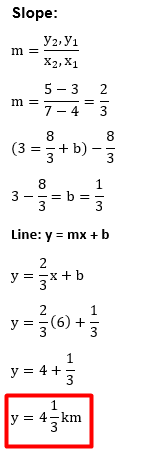# Scatter Plots and Lines of Best Fit Worksheet 1 (With answer key +PDF)

A scatter plot is a graph that displays ordered pairs of data. If two sets of data are related or associated with one another, you can use a scatter plot to show this. A correlation is another name for this. In a scatter plot, a line of best fit is a straight line that accurately depicts the data.

## What is the “Scatter Plots and Lines of Best Fit Worksheet 1 (With answer key +PDF)”?

This worksheet will explore some of the scatter plots and lines of best fit.

What are scatter plots and lines of best fit?

The term “line of best fit” describes a line that passes through a scatter plot of data points and best captures their relationship. Points on a scatter (XY) plot depict how two sets of data relate to one another.

How will the “Scatter Plots and Lines of Best Fit Worksheet 1 (With answer key +PDF)” help you?

This worksheet will aid in your understanding of the specified subject, and students will be able to respond to the exercises provided below.

Instructions on how to use the “Scatter Plots and Lines of Best Fit Worksheet 1 (With answer key +PDF).”

Study the concept and examples given and try to solve the given exercises below.

Conclusion

To make inferences about the data, use the best fit lines. Make use of scatterplots to gather, display, and interpret data. To estimate a line of best fit and come up with an equation for the line, use the scatterplot’s shape as a guide.

If you have any inquiries or feedback, please let us know.

## Scatter Plots and Lines of Best Fit Worksheet 1 (With answer key +PDF)

A scatter plot is a tool for graphically presenting data. A straightforward scatter plot uses the coordinate axes to plot the points according to their values.

Example: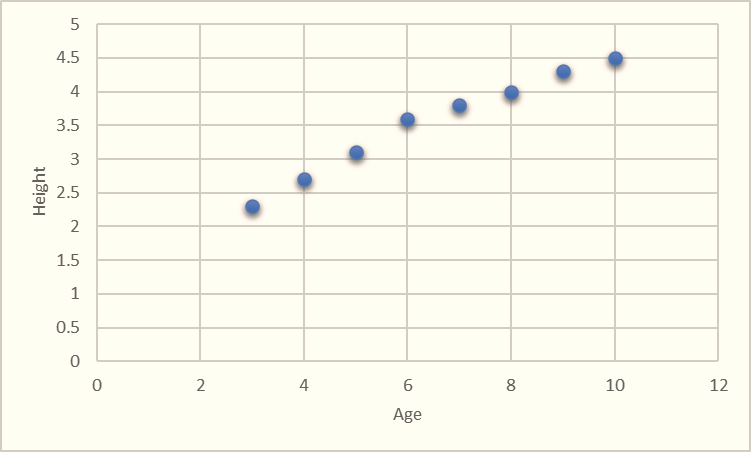Finding the relationship between two variables is assisted by a scatter plot. A correlation is a term used to describe this connection. Based on the correlation, scatter plots can be classified as follows.

• Scatter Plot for Positive Correlation
• Scatter Plot for Negative Correlation
• Scatter Plot for Null Correlation

line of best fit is a straight line that is the best approximation of the given set of data. A line of best fit can be roughly determined using an eyeball method by drawing a straight line on a scatter plot so that the number of points above the line and below the line is about equal (and the line passes through as many points as possible). A more accurate way of finding the line of best fit is the least square method.

Step 1: Calculate the mean of the x -values and the mean of the y -values.

Step 2: The following formula gives the slope of the line of best fit: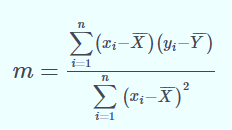Step 3: Compute the y-intercept of the line by using the formula: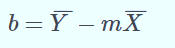Step 4: Use the slope m and the y-intercept b to form the equation of the line.

Example: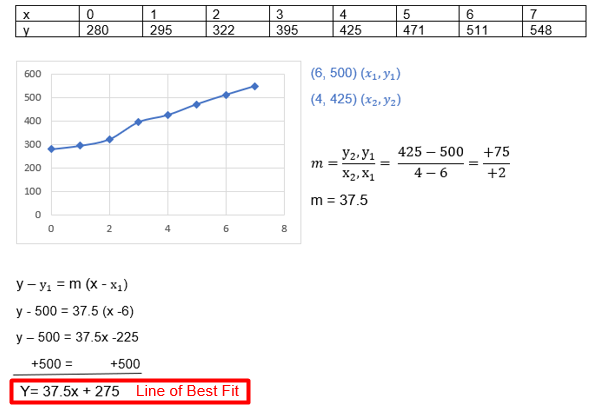## Worksheet

1. A group of college students wore fitness trackers. The scatter plot below represents the relationship between the number of active hours they walked, x, versus the distance, they traveled, y. Write an equation that approximates the lines of best fit. Predict the distance in kilometers a college student will walk in 6 hours. When (4,3).(x1,y1)and (7,5) ( x2,y2).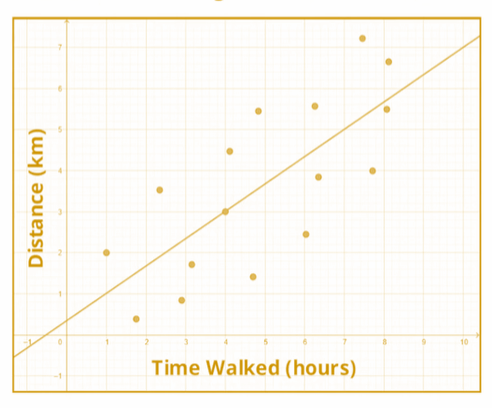1. Reflection

What do you think about solving the Scatter Plots and Lines of Best Fit? Did you find it difficult to follow the pattern in each step?